# Constant Pressure ProcessFor a mass of gas consisting of n= moles at a pressure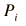= kPa = x10^ Pa,

the expansion from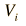= m3
 to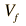= m3

involves an amount of work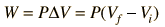= J = x10^ J

In order to accomplish this work, energy must be added, and to calculate this energy, the temperatures must be determined. They can be calculated from the pressure and volumes using the ideal gas law: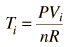= K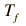= K
 Then if the molar specific heat is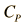= J/mole K

the amount of heat required is given by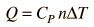= J = x10^ J
Index

Heat engine concepts

 HyperPhysics***** Thermodynamics R Nave
Go Back